×

Search anything:

# Move Last Element of Linked List to FrontFREE BOOK -> Problems for the day before your Coding Interview (on Amazon)

We will explore recursive and iterative algorithm to Move Last Element of Linked List to Front.

# Introduction

A linked list is a collection of nodes where each node is connected to the next node through a pointer. The first node is called a head and if the list is empty then the value of head is NULL.

Each node in a list consists of at least two parts:

• Data
• Pointer to the next node

### Representation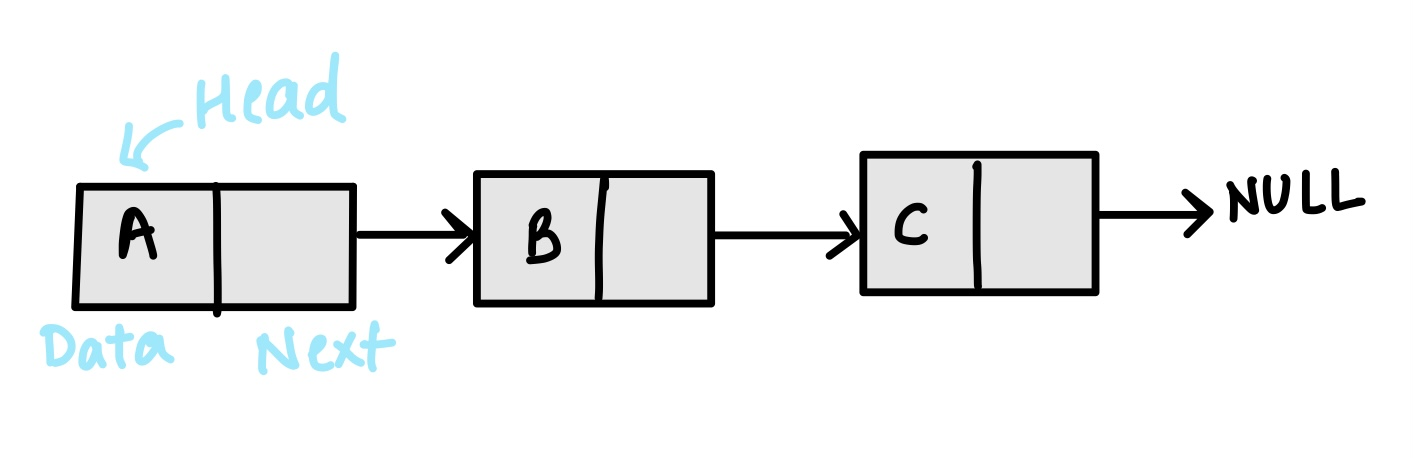1. Singly Linked List: Singly linked lists contain nodes which have a data field as well as 'next' field, which points to the next node in line of nodes. Operations that can be performed on singly linked lists include insertion, deletion and traversal.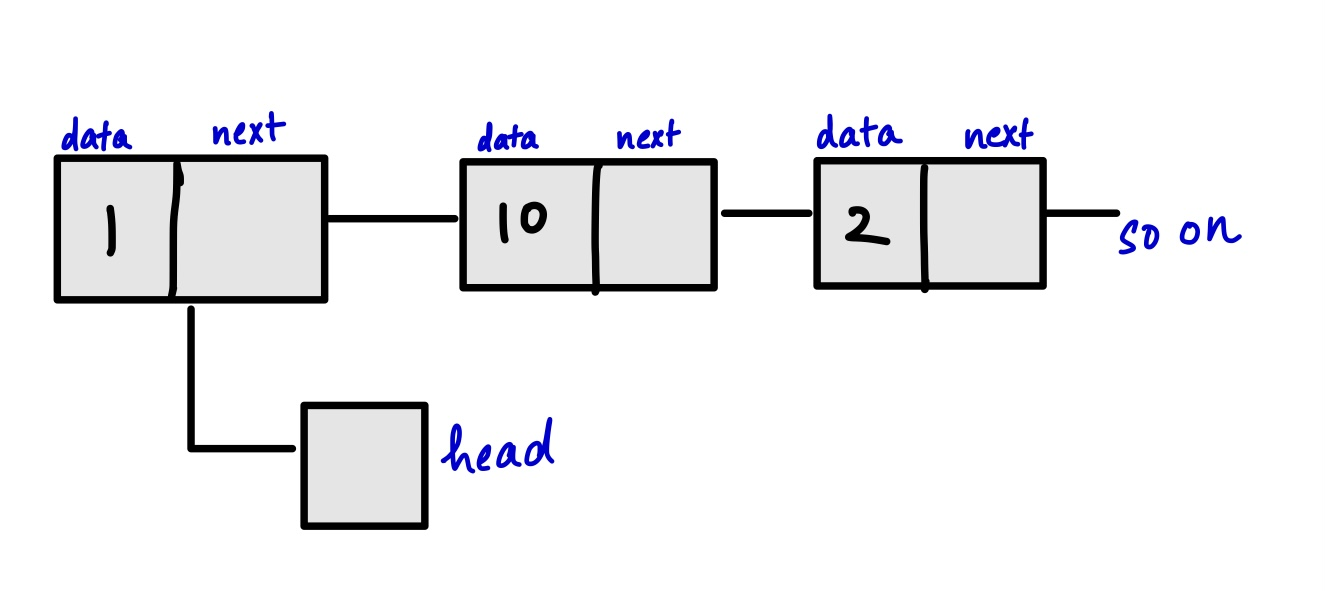1. Doubly Linked List: Each node contains, besides the next-node link, a second link field pointing to the 'previous' node in the sequence. The two links may be called forward and backwards, or next and previous.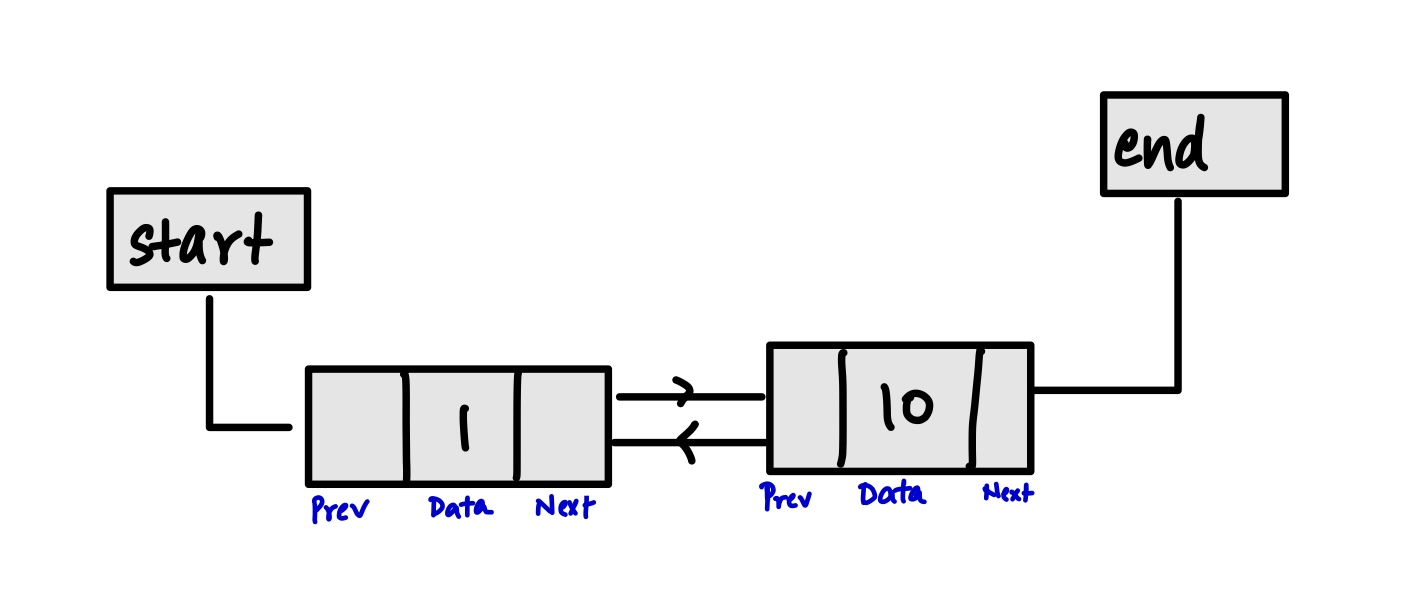1. Circular Linked List: In the last node of a list, the link field often contains a null reference, a special value is used to indicate the lack of further nodes. It is a list where the last pointer points to the first node.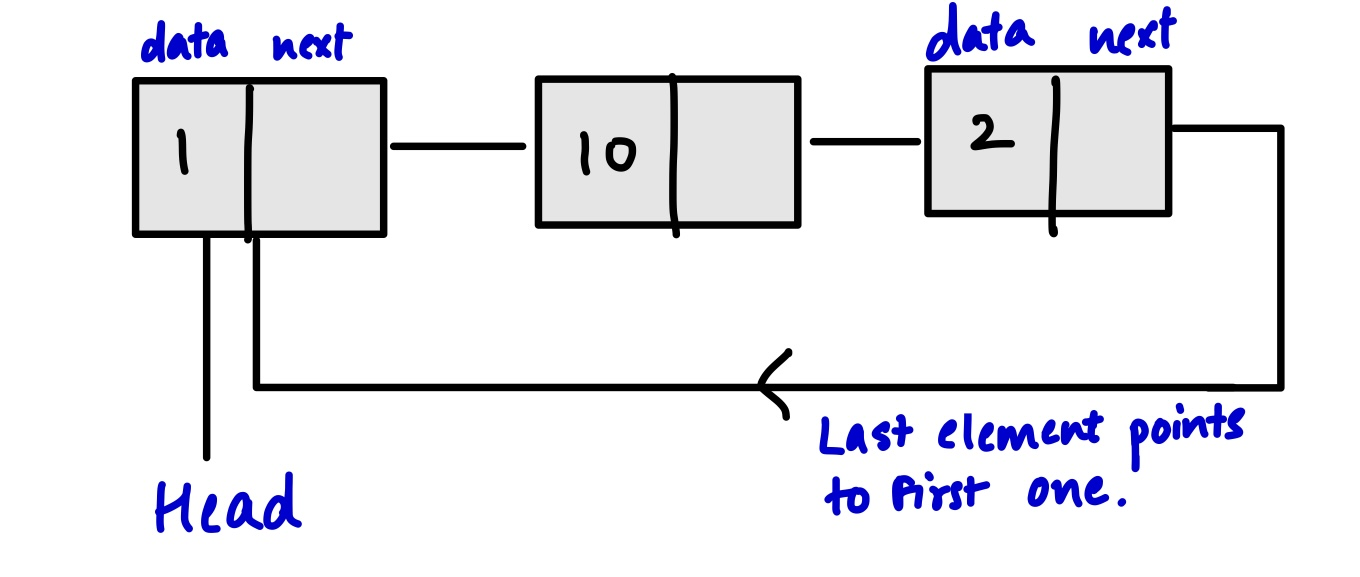1. Multiply linked list : Each node contains two or more link fields, each field being used to connect the same set of data sets in a different order of same set(e.g., by name, by department, by date of birth, etc.).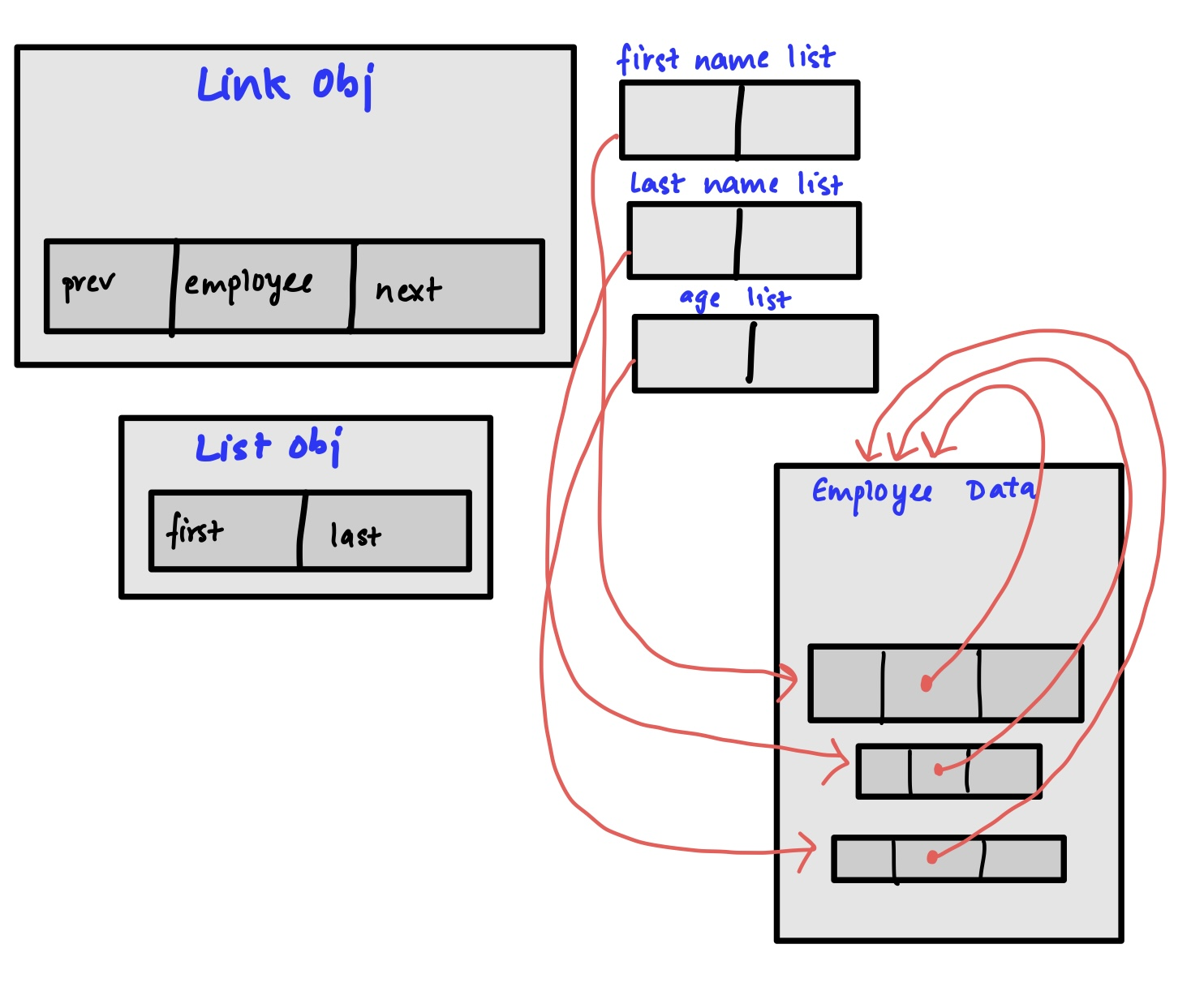• Has a dynamic data structure.
• No memory wastage and no need to pre-allocate the memory.
• Insertion and Deletion of operations is done easily.
• Stacks & Queues can be eaily implemented.
• Size is not fixed
• Can be extended even reduced according to the requirement needed .

• Random access is not possible due to dynamic memory allocation.
• Traversal is more time-consuming .We can not traverse it from last and only from the beginning.
• More memory is required .
• Time is acquired to access each element present .
• Not very easy to sort the elements present in the Linked List.

• Linked lists are used to implement stacks, queues, graphs, etc.
• Linked lists let you insert elements at the beginning and end of the list.
• In Linked Lists we don't need to know the size in advance.
• Implementing Hash Tables
• Accessing next element in a Linked List takes less time as compared to an Array. This is because Linked List takes the advantage of locality of reference
• Manipulation of polynmials by storing constant numbers in the node
• Sparse Matrices Representation .
• Implementation of graphs.

Now we will talk about the implementation with logical approach for how to move the last element of Linked List to first/front position .

### IMPLEMENTATION

ALGORITHM
Step 1 : create a function which takes linked list as argument and gives a new linked list with last element in front.
Step 2 : Traverse till last node.
Store both last and second last node.
Step 3 : Make the second last node as last node
make the next of second last as NULL as after moving it will become the last node.
Step 4 : Make next of last as head.
Step 5 : Make the last node the head.
This is done by giving changing the head pointer to last.
Step 6 : call the function on given linked list. It will print a new linked list with last as head.

Let's take an example :
If the given linked list is 1->2->3->4
Then, the output is 4->1->2->3

### Algorithm (Theory approach step-by-step):

1. Firstly, traverse the linked list till the last node.
2. Secondly, we will use two pointers here.
3. Now, use one pointer to store the address of the last node and second pointer to store the address of the second last node.
4. Do following after the end of the loops.
5. Now make the second last node as the last node.
6. Then set next of the last node as head of the list.
7. Finally, make the last node as the head of the list.
8. The time complexity of the solution is O(n ) where s is the total number of nodes in the list.

The pseudo code will be like this :

``````void moveLastToFront(node **root) {
node* prev = NULL;
node* last = *root;

while( last->next != NULL) {
prev = ptr;
prev = last->next;
}
prev->next = NULL;
last->next = *root;
*root = last;

}
``````

### Logical Approach

We have to move last element of Linked List to front

Example list {1,2,3,4} should be changed to {4,1,2,3}

In JAVA

``````// A Linked List Node
class Node
{
int data;
Node next;

Node(int data, Node next) {
this.data = data;
this.next = next;
}
}

class Main
{
// Helper function to print a given linked list
{
while (ptr != null)
{
System.out.print(ptr.data + " —> ");
ptr = ptr.next;
}

System.out.println("null");
}

// Function to move the last node to the front of a given linked list
{
// proceed only when the list is valid
return null;

// move to the second last node
while (ptr.next.next != null) {
ptr = ptr.next;
}

// transform the list into a circular list

// break the chain
ptr.next= null;

}

public static void main(String[] args)
{
// input keys
int[] keys = { 1, 2, 3, 4 };

for (int i = keys.length - 1; i >= 0; i--) {
}

}
}
``````

In JAVA (RECURSIVE)

``````// A Linked List Node
class Node
{
int data;
Node next;

Node(int data, Node next) {
this.data = data;
this.next = next;
}
}

class Main
{
// Helper function to print a given linked list
{
while (ptr != null)
{
System.out.print(ptr.data + " —> ");
ptr = ptr.next;
}

System.out.println("null");
}

// Recursive function to move the last node to the front of a given linked list
public static Node rearrange(Node head, Node prev, Node curr)
{
// if the current node is the last
if (curr.next == null)
{
// make its next point to the first node

// set its previous node to point to null
prev.next = null;

// return current reference (new head)
return curr;
}

}

// Function to move the last node to the front of a linked list
{
// if the list contains at least two nodes
}

}

public static void main(String[] args)
{
// input keys
int[] keys = { 1, 2, 3, 4 };

for (int i = keys.length - 1; i >= 0; i--) {
}

}
}

``````

Output
4 -> 1 -> 2 -> 3

### TIME COMPLEXITY

Given time complexity is O(n) where 'n' is number of nodes present in Linked List.

Learn at OpenGenus IQ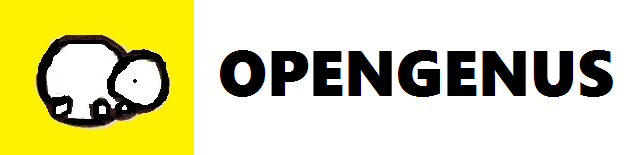#### Prnika Bakshi

Intern at OpenGenus; Pursuing B. Tech in Information Technology; a DSC lead and a Microsoft Learn Student Ambassador;

Move Last Element of Linked List to Front